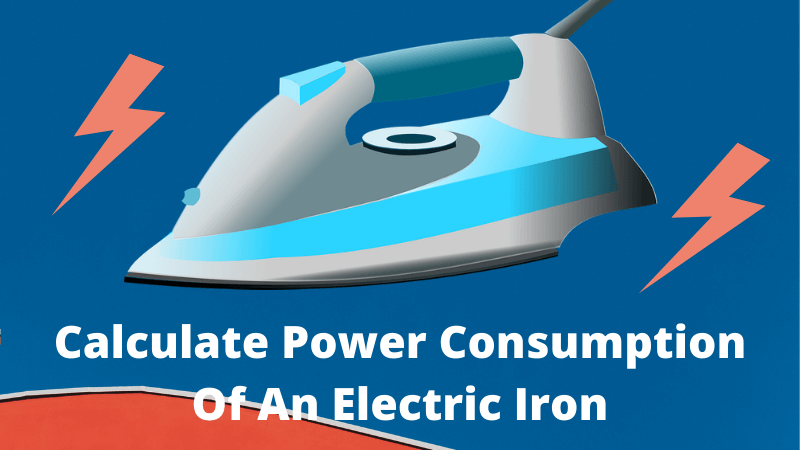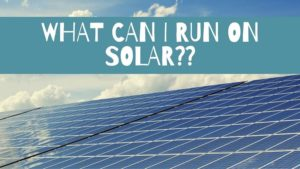## Calculate Power Consumption of an Electric Iron:Electric iron is a common household appliance used to remove wrinkles and creases out of clothes and make them more presentable. Power consumption of an electric iron or for that matter any appliance depends on its rated power and operational hours.

On an average most electric iron has wattage between 1000 watts to 2000 watts. If you use a 1000 watt electric iron for 10 minutes everyday for an entire month it will consume 5 kWh of electricity.

On an average the rated power of an electric iron is somewhere between 1000 watts to 2000 watts, which is way higher than majority of the household appliances.

The most commonly used electric iron has power wattage of 1000 watts, you can check the label on your electric iron to get the exact wattage.

To use the electric iron power consumption calculator to calculate the power consumption of your electric iron and interpret the results properly you should understand the following terms.

• What is Watt (W) – Watt is a unit of power. It means the rate at which electricity is consumed or produced by a device. For example a 50 watt TV consumes power at a rate of 50 watts per hour, it does not mean that the TV consumed 50 units of electricity, it means it will consume power at a rate of 50 watts every hour.
• What is Kilowatt (kW) – Kilowatt is also a unit of power. Kilowatt (KW) is used for large devices that consume more power. For example a 1.5 kW air conditioner, 6 kW elevator.
• What is a Kilowatt hour (kWh) – Units as described in electricity bill a.k.a kilowatt hour is the energy consumption of a device. For example a 50 watt table fan running for 50 hours will consume 50 watt x 50 hours = 2500 watt hours = 2.5 kilowatt hours of electricity = 2.5 units of electricity. (1 kWh of electricity = 1 unit of electricity)
• Electricity Tariff – Electricity tariff is the amount the electricity provider charges you for one unit (kWh) of electricity. I live in Mumbai, India here the electricity tariff is Rs 12/kWh. If you don’t know how to calculate your tariff then just divide your total bill by monthly power consumption and you will get your electricity tariff.
• What is operational hours – Operational hours of a device refers to how many hours the device is working. If you run your television for 3 hours then operational hours of your television is 3 hours.

To calculate the power consumption of your electric iron Just put the wattage of your electric iron, operational hours and electricity tariff of your locality and you will get the units of electricity consumed by your electric iron and the impact it has on your electricity bill

## Electric Iron Power Consumption Calculator:

How To Interpret the calculated result:

The power consumed by your electric iron is actually less than what the calculator shows. The reason for that is, if you have to iron bunch of clothes and you know it will take 30 minutes to do it you don’t keep the electric iron turned ON for the whole 30 minutes you turn it ON and once the bottom plate of the iron is hot you turn OFF the power supply.

When the bottom plate of the iron becomes cold and you can see that it cannot iron your clothes properly you turn ON the power supply to get the plate heated up.

I have analysed that whenever we iron our clothes the electric iron is turned ON for maximum 50% of the time and for the rest of the time it is OFF but the heated plate gets the job done.

So whatever value you are getting in the calculator just divide it by 2 to get the actual power consumption of your electric iron.

#### Check out related blogs:## Best Free Ways To Save Electricity Bill Of Your House## What Can I Run On Solar?

Can I run my air conditioner, refrigerator, water pump on...